Скачать презентацию PHYS 1444 Section 004 Lecture 18 Wednesday

3bce8e00669aa8ffa4502184f5e8d057.ppt

• Количество слайдов: 14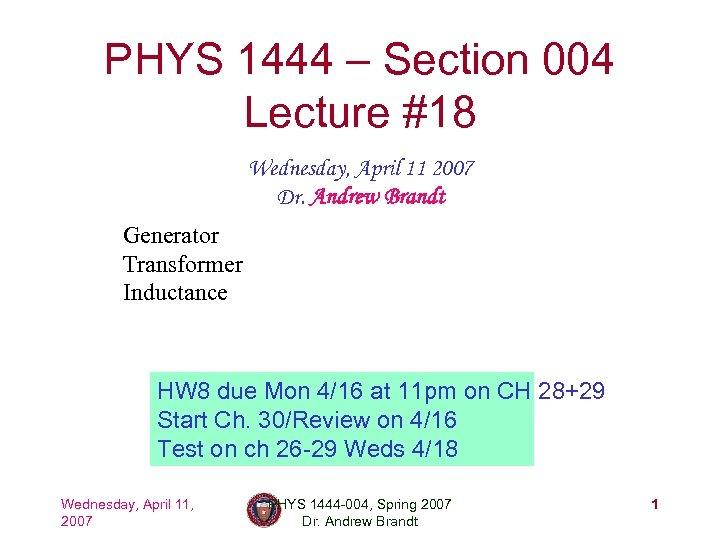PHYS 1444 – Section 004 Lecture #18 Wednesday, April 11 2007 Dr. Andrew Brandt Generator Transformer Inductance HW 8 due Mon 4/16 at 11 pm on CH 28+29 Start Ch. 30/Review on 4/16 Test on ch 26 -29 Weds 4/18 Wednesday, April 11, 2007 PHYS 1444 -004, Spring 2007 Dr. Andrew Brandt 1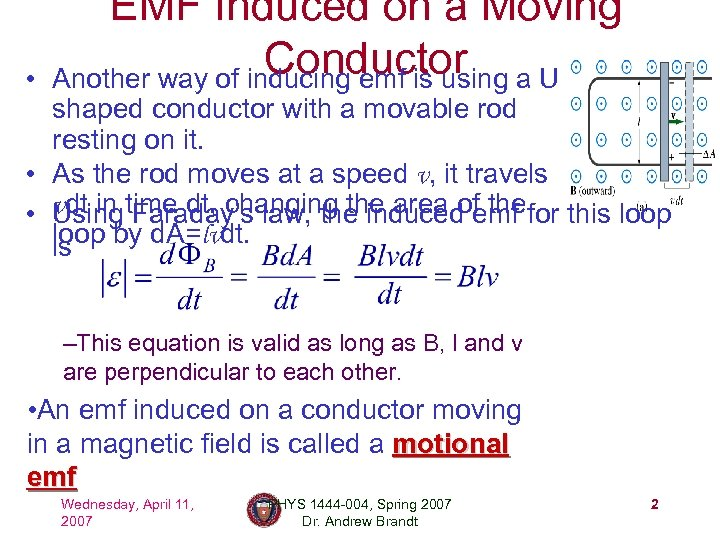• EMF Induced on a Moving Conductor a U Another way of inducing emf is using shaped conductor with a movable rod resting on it. • As the rod moves at a speed v, it travels • vdt in time dt, changing the area of thefor this loop Using Faraday’s law, the induced emf loop by d. A=lvdt. is –This equation is valid as long as B, l and v are perpendicular to each other. • An emf induced on a conductor moving in a magnetic field is called a motional emf Wednesday, April 11, 2007 PHYS 1444 -004, Spring 2007 Dr. Andrew Brandt 2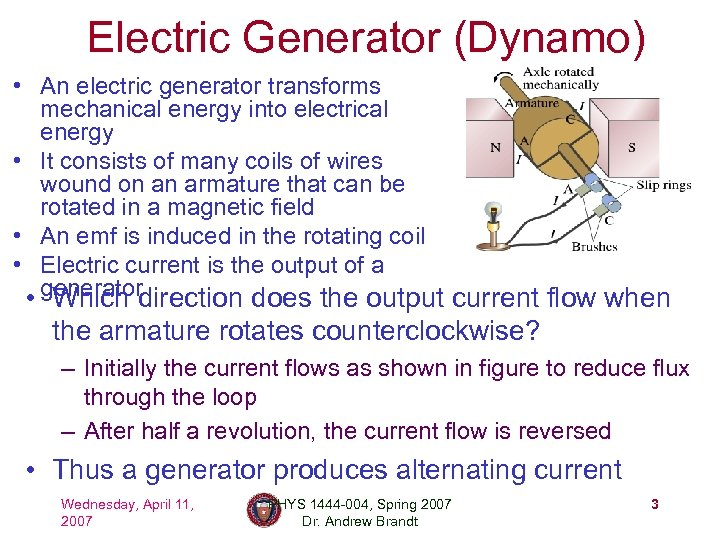Electric Generator (Dynamo) • An electric generator transforms mechanical energy into electrical energy • It consists of many coils of wires wound on an armature that can be rotated in a magnetic field • An emf is induced in the rotating coil • Electric current is the output of a • generatordirection does the output current flow when Which the armature rotates counterclockwise? – Initially the current flows as shown in figure to reduce flux through the loop – After half a revolution, the current flow is reversed • Thus a generator produces alternating current Wednesday, April 11, 2007 PHYS 1444 -004, Spring 2007 Dr. Andrew Brandt 3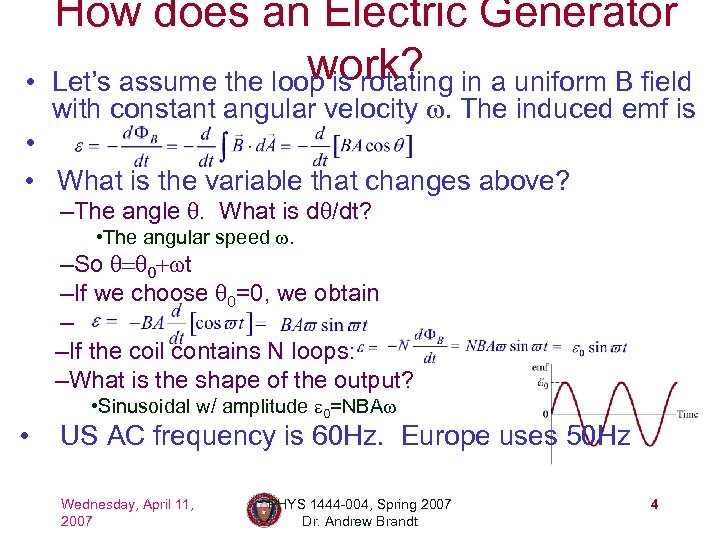• How does an Electric Generator work? Let’s assume the loop is rotating in a uniform B field with constant angular velocity w. The induced emf is • • What is the variable that changes above? –The angle q. What is dq/dt? • The angular speed w. –So q=q 0+wt –If we choose q 0=0, we obtain – –If the coil contains N loops: –What is the shape of the output? • • Sinusoidal w/ amplitude e 0=NBAw US AC frequency is 60 Hz. Europe uses 50 Hz Wednesday, April 11, 2007 PHYS 1444 -004, Spring 2007 Dr. Andrew Brandt 4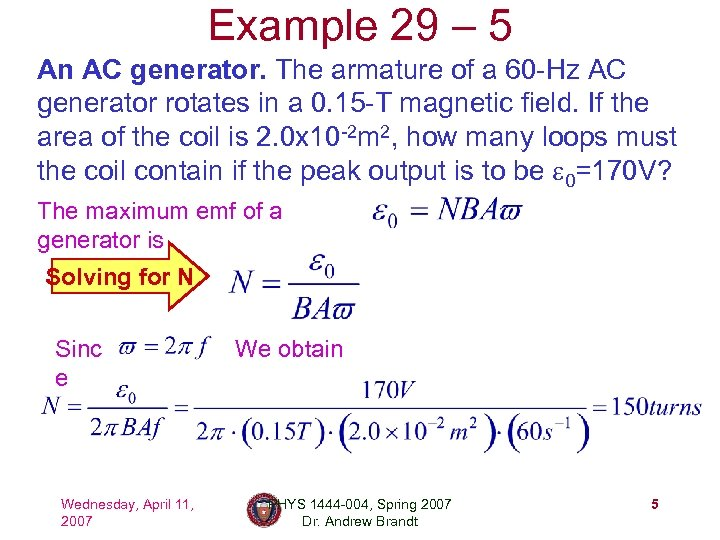Example 29 – 5 An AC generator. The armature of a 60 -Hz AC generator rotates in a 0. 15 -T magnetic field. If the area of the coil is 2. 0 x 10 -2 m 2, how many loops must the coil contain if the peak output is to be e 0=170 V? The maximum emf of a generator is Solving for N Sinc e Wednesday, April 11, 2007 We obtain PHYS 1444 -004, Spring 2007 Dr. Andrew Brandt 5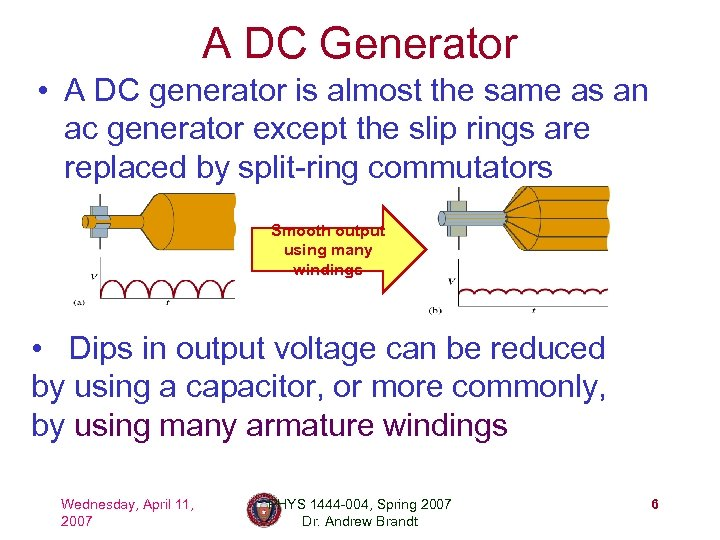A DC Generator • A DC generator is almost the same as an ac generator except the slip rings are replaced by split-ring commutators Smooth output using many windings • Dips in output voltage can be reduced by using a capacitor, or more commonly, by using many armature windings Wednesday, April 11, 2007 PHYS 1444 -004, Spring 2007 Dr. Andrew Brandt 6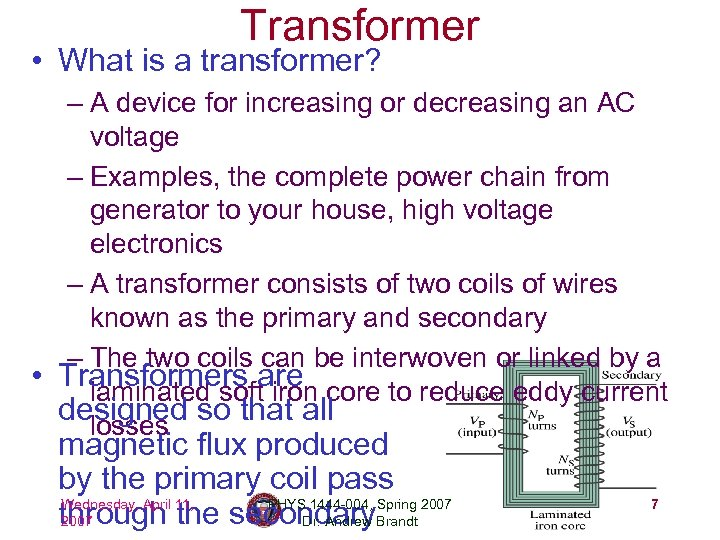Transformer • What is a transformer? – A device for increasing or decreasing an AC voltage – Examples, the complete power chain from generator to your house, high voltage electronics – A transformer consists of two coils of wires known as the primary and secondary – The two coils can be interwoven or linked by a • Transformers are core to reduce eddy current laminated soft iron designed so that all losses magnetic flux produced by the primary coil pass Wednesday, April 1444 -004, through 11, secondary. Brandt 2007 the PHYSDr. Andrew Spring 2007 7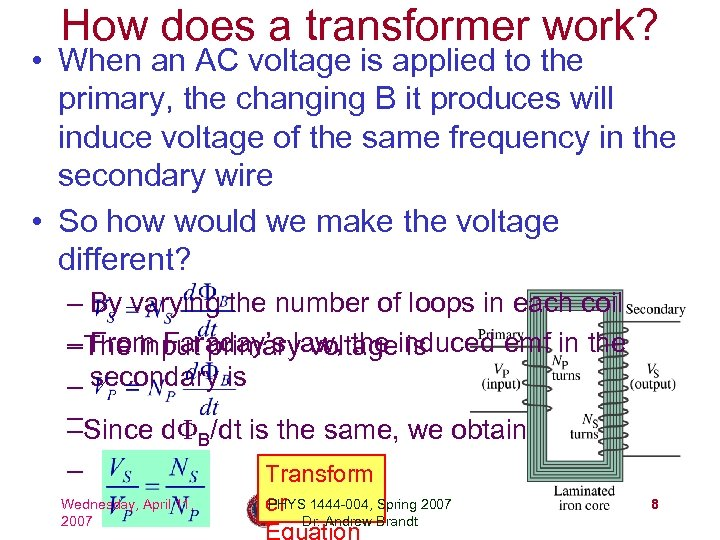How does a transformer work? • When an AC voltage is applied to the primary, the changing B it produces will induce voltage of the same frequency in the secondary wire • So how would we make the voltage different? – By varying the number of loops in each coil –The input primary voltageinduced emf in the From Faraday’s law, the is – secondary is –Since d. F /dt is the same, we obtain – B – Wednesday, April 11, 2007 Transform PHYS er 1444 -004, Spring 2007 Dr. Andrew Brandt Equation 8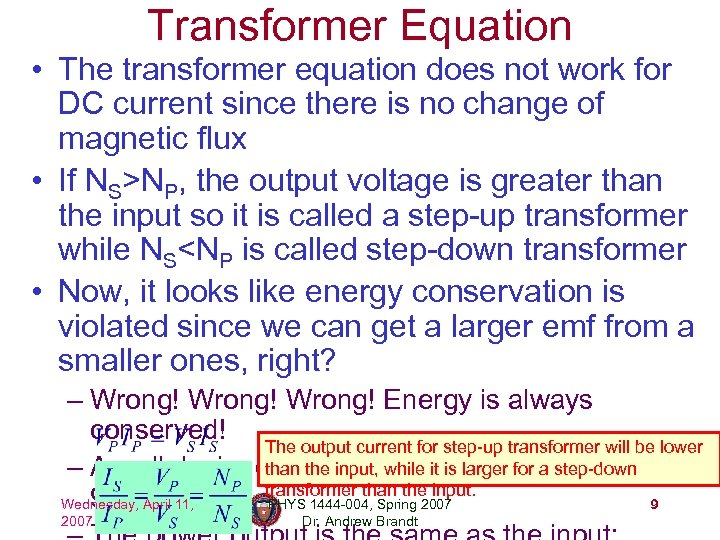Transformer Equation • The transformer equation does not work for DC current since there is no change of magnetic flux • If NS>NP, the output voltage is greater than the input so it is called a step-up transformer while NS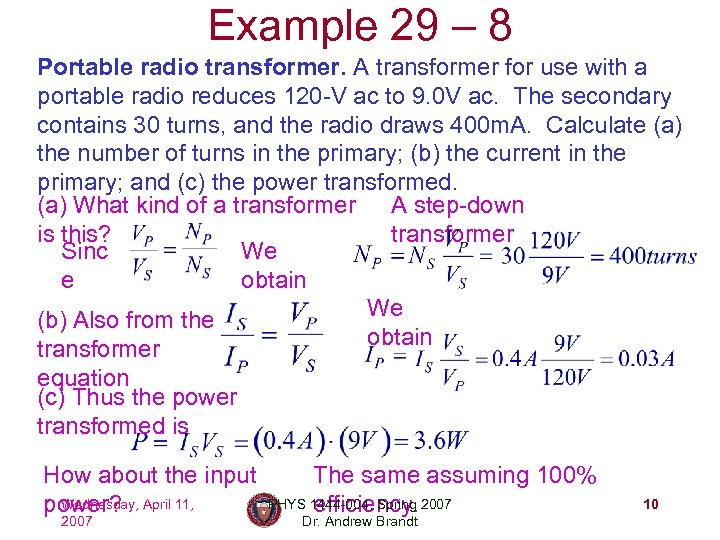Example 29 – 8 Portable radio transformer. A transformer for use with a portable radio reduces 120 -V ac to 9. 0 V ac. The secondary contains 30 turns, and the radio draws 400 m. A. Calculate (a) the number of turns in the primary; (b) the current in the primary; and (c) the power transformed. (a) What kind of a transformer A step-down is this? transformer Sinc We e obtain We (b) Also from the obtain transformer equation (c) Thus the power transformed is How about the input Wednesday, power? April 11, 2007 The same assuming 100% PHYS 1444 -004, Spring 2007 efficiency. Dr. Andrew Brandt 10Example 29 – 9: Power Transmission of electric Transmission lines. An average of 120 k. W power is sent to a small town from a power plant 10 km away. The transmission lines have a total resistance of 0. 4 W. Calculate the power loss if the power is transmitted at (a) 240 V and (b) 24, 000 V. We cannot use P=V 2/R since we do not know the voltage along the transmission line. We, however, can use P=I 2 R. (a) If 120 k. W is sent at 240 V, the total current the power loss due to the Thus is transmission line is (b) If 120 k. W is sent at 24, 000 V, the total current the power loss due to Thus is transmission line is The higher the transmission voltage, the smaller the current, causing less Wednesday, April 11, PHYS 1444 -004, Spring 2007 11 loss of energy. This is why power is transmitted w/ HV, as high as 170 k. V. 2007 Dr. Andrew Brandt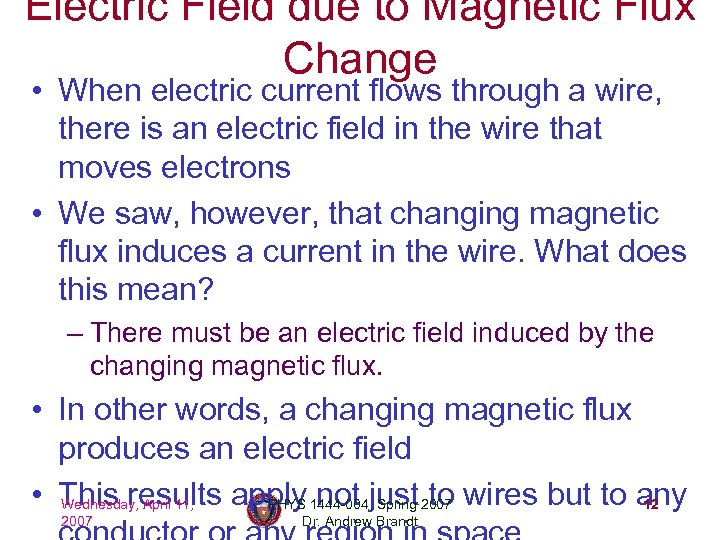Electric Field due to Magnetic Flux Change • When electric current flows through a wire, there is an electric field in the wire that moves electrons • We saw, however, that changing magnetic flux induces a current in the wire. What does this mean? – There must be an electric field induced by the changing magnetic flux. • In other words, a changing magnetic flux produces an electric field • This results apply 1444 -004, just 2007 wires but to any Wednesday, April 11, PHYS not Spring to 12 2007 Dr. Andrew Brandt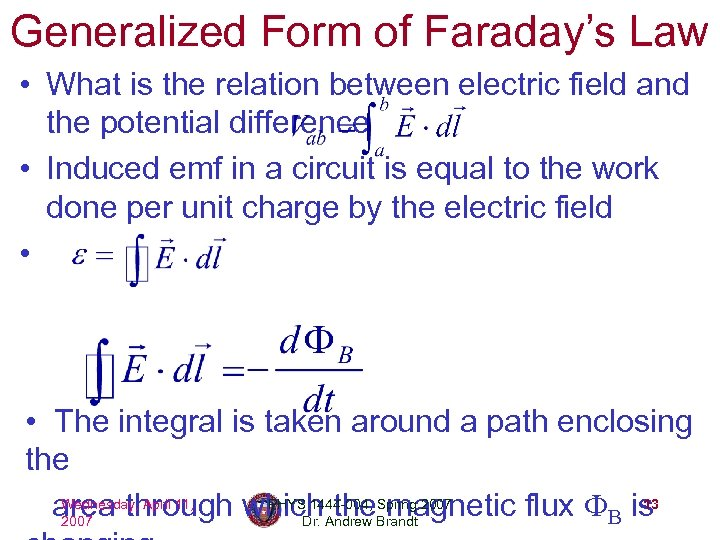Generalized Form of Faraday’s Law • What is the relation between electric field and the potential difference • Induced emf in a circuit is equal to the work done per unit charge by the electric field • • The integral is taken around a path enclosing the Wednesday, April 11, PHYS 1444 -004, Spring 13 area through which Andrew Brandt 2007 the magnetic flux FB is 2007 Dr.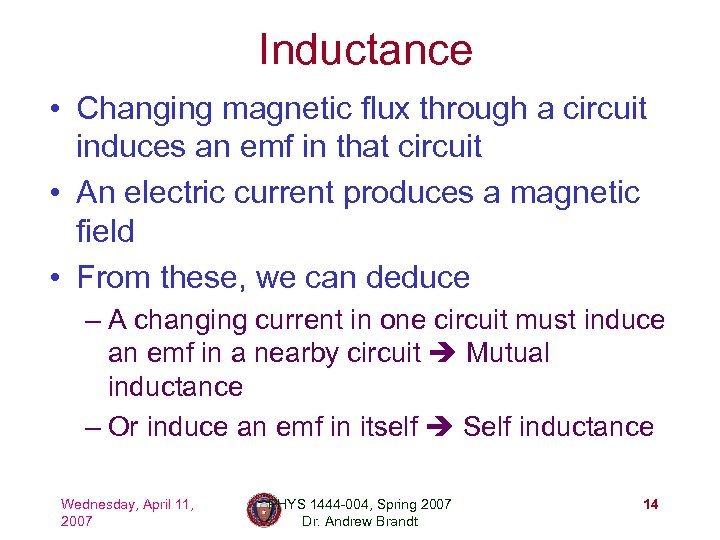Inductance • Changing magnetic flux through a circuit induces an emf in that circuit • An electric current produces a magnetic field • From these, we can deduce – A changing current in one circuit must induce an emf in a nearby circuit Mutual inductance – Or induce an emf in itself Self inductance Wednesday, April 11, 2007 PHYS 1444 -004, Spring 2007 Dr. Andrew Brandt 14